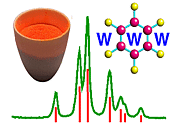Symmetry Groups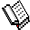Course Material Index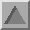Section Index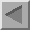Previous Page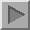Next Page

Concepts

Before providing a formal definition of both a group and a point group, we will give an example of a group, namely, the point group 6. The earlier section on rotational symmetry demonstrated that the sixfold rotation operator rotated a benzene ring to equivalent positions when applied to the sixfold rotation axis. The point group 6 contains the following symmetry operators:

6+    3+    2     3-    6-    1

which correspond to anticlockwise rotations of 60°, 120°, 180°, 240° (or -120°), 300° (or -60°), and 360° (or 0°), respectively, about the sixfold rotation axis. (Remember that the rotation axis is the symmetry element and not the symmetry operator). These 6 symmetry operators form a group according to the rules given below.

A symmetry group is a set of symmetry operators that are combined together according to a particular set of rules:

• The resulting action from the combination of two symmetry operators must always be equivalent to the action of a single symmetry operator that belongs to the same group.

With reference to the point group 6, the action of a 60° rotation followed by, say, a 240° rotation is equivalent to a rotation of 300°. In symbol form this is written as:

6+  *   3-  =    6-
Thus the rule is obeyed since the resulting operator (6-) is a member of the point group 6.

• The group must always contain the identity symmetry operator.

Again with reference to the point group 6, this is the rotation axis of order 1 which rotates an object by 360°.

• Each symmetry operator in the group must have an inverse operator belonging to the group.

Thus in the point group 6, the inverse operator for a positive 60° rotation (6+) is a negative rotation (6-) by 60°.

• Beyond the scope of this course is the associative rule specifying the way in which symmetry operators are combined with each other.

A point group may be defined as any group of symmetry operators that leaves one or more points in space unmoved. If it is a single point, it is fixed in space by (a) the centre of inversion when the point group posseses rotary-inversion axes (e.g −1, −3, −4, −5, −6, −7, ..., −∞), or (b) the intersection of a twofold (2) and one or more axes (e.g. 2, 3, 4, 5, 6, 7, ..., ∞) or (c) the intersection of a mirror plane (m) and a rotation axis (e.g. 2, 3, 4, 5, 6, 7, ..., ∞) perpendicular to it. This single fixed point is called the origin. If it involves more than one point unmoved, then the point group is polar as discussed later under the subject of polar point groups.

Although there exists an infinite number of point groups, when crystallographic considerations are taken into account, i.e. rotation and rotary-inversion axes must be of order 1, 2, 3, 4, or 6, then the number of such groups is limited to just 32. The symmetry operators present in these groups are:

 Rotation Axes Rotary-Inversion Axes 1 2 3 4 6 -1 m (= -2) -3 -4 -6

The 32 point groups consistent with the 7 crystal systems are known as the 3-dimensional crystallographic point groups.Course Material IndexSection IndexPrevious PageNext Page
 © Copyright 1995-2006.  Birkbeck College, University of London. Author(s): Jeremy Karl Cockcroft Huub Driessen David Moss